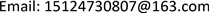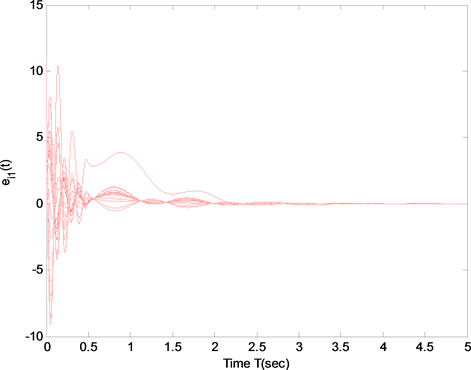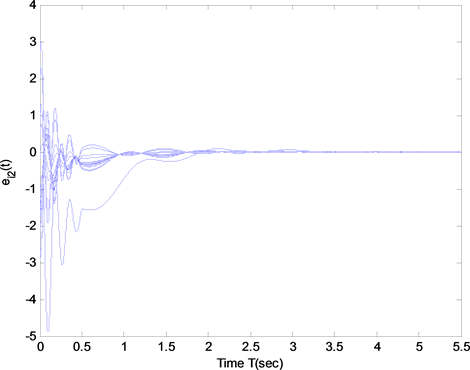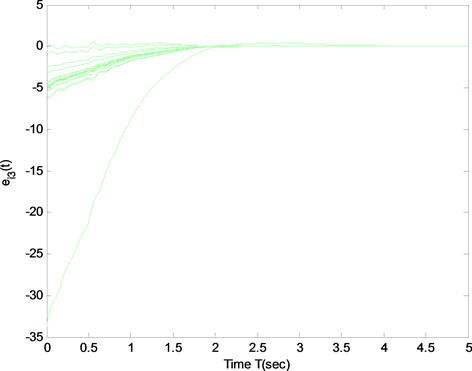﻿ 具有节点时滞的复杂网络的同步和局部收敛性分析 Synchronization and Local Convergence Analysis of Complex Dynamical Networks with Nodes Delay

Operations Research and Fuzziology
Vol.08 No.02(2018), Article ID:25164,8 pages
10.12677/ORF.2018.82007

Synchronization and Local Convergence Analysis of Complex Dynamical Networks with Nodes Delay

Jingwen Mu, Caixia Gao

School of Mathematical Science, Inner Mongolia University, Hohhot Inner MongoliaReceived: May 9th, 2018; accepted: May 21st, 2018; published: May 29th, 2018ABSTRACT

In recent years, synchronization of complex networks has received an increasing attention due to their wide applications in many fields. To analyze and reveal the inherent mechanism of local synchronization in the complex networks with delayed nodes, this paper attempts to investigate it by the Lyapunov direct method which can be utilized as a theoretical methodology. First, we investigated local synchronization of complex networks with delayed nodes by this method and obtained some sufficient conditions to guarantee the networks reaching local synchronization. The criterion is very simple and can be readily applied in the real world networks. Then, an example is provided to verify the effectiveness of the main results.

Keywords:Synchronization, Local Convergence, Complex Networks, Delayed Nodes, Lyapunov Direct MethodCopyright © 2018 by authors and Hans Publishers Inc.1. 引言

2. 预备知识

$\stackrel{˙}{x}\left(t\right)=f\left({x}_{i}\left(t\right),{x}_{i}\left(t-\tau \right)\right)+\underset{j=1,j\ne i}{\overset{m}{\sum }}{a}_{ij}\Gamma \left({x}_{j}\left(t\right)-{x}_{i}\left(t\right)\right)$ (1)

$\underset{t\to \infty }{\mathrm{lim}}‖{x}_{i}\left(t,\tau ;{t}_{0},{X}_{0}\right)-s\left(t,\tau ;{t}_{0},{X}_{0}\right)‖=0$

1) ${a}_{ij}\ge 0,\left(i\ne j\right),\text{\hspace{0.17em}}{a}_{ii}=-{\sum }_{j=1,j\ne i}^{N}{a}_{ij}\left(i=1,2,\cdots ,m\right)$

2) A的特征值均具有负实部，除了单特征值0。

1) ${a}_{ij}\ge 0,\left(i\ne j\right),\text{\hspace{0.17em}}{a}_{ii}=-{\sum }_{j=1,j\ne i}^{N}{a}_{ij}\left(i=1,2,\cdots ,m\right)$

2) A是不可约的。

1) 若λ是A的特征值， $\lambda \ne 0$ ，则 $\mathrm{Re}\left(\lambda \right)<0$

2) A必有一个单特征值 $0$ ，且其对应的右特征向量为 ${\left[1,1,\cdots ,1\right]}^{\text{T}}$

3) 假设 $\xi ={\left[{\xi }_{1},{\xi }_{2},\cdots ,{\xi }_{m}\right]}^{\text{T}}\in {R}^{m}$ (不失一般性，假设 ${\sum }_{i=1}^{m}{\xi }_{i}=1$ )是A对应于特征值0的左特征向量，则对所有的 $i=1,2,\cdots ,m$ ，均有 ${\xi }_{i}\ge 0$

4) 若 $A\in {A}_{2}$ ，则对所有的 $i,i=1,2,\cdots ,m$ ，当且仅当 ${\xi }_{i}>0$ 成立。

5) 若A是可约的，当且仅当存在某些下标i，有 ${\xi }_{i}=0$ 。此时，经过适当的置换变换，可假设 ${\xi }^{\text{T}}=\left[{\xi }_{+}^{\text{T}},{\xi }_{0}^{\text{T}}\right]$ ，其中 ${\xi }_{+}={\left[{\xi }_{1},{\xi }_{2},\cdots ,{\xi }_{p}\right]}^{\text{T}}\in {R}^{p}$ 满足 ${\xi }_{i}>0,i=1,2,\cdots ,p\left(p ，且

${\xi }_{0}={\left[{\xi }_{p+1},{\xi }_{p+2},\cdots ,{\xi }_{m}\right]}^{\text{T}}\in {R}^{m-p}$ 满足 ${\xi }_{j}=0$$j=p+1,p+2,\cdots ,m$ 。则A可分解成 $\left[\begin{array}{cc}{A}_{11}& {A}_{12}\\ {A}_{21}& {A}_{22}\end{array}\right]$ ，其中 ${A}_{11}\in {R}^{p,p}$ 是不可约的，且 ${A}_{12}=0$

3. 主要结果

${\stackrel{˙}{x}}_{i}=f\left({x}_{i}\left(t\right),{x}_{i}\left(t-\tau \right)\right)+\underset{j=1}{\overset{m}{\sum }}{a}_{ij}\Gamma {x}_{j}\left(t\right)$ (2)

${\stackrel{˙}{x}}_{i}=f\left({x}_{i}\left(t\right),{x}_{i}\left(t-\tau \right)\right)-\underset{j=1}{\overset{m}{\sum }}{l}_{ij}\Gamma {x}_{j}\left(t\right)$ (3)

$\begin{array}{c}\frac{\text{d}\stackrel{¯}{x}\left(t\right)}{\text{d}t}=\underset{i=1}{\overset{m}{\sum }}{\xi }_{i}\frac{\text{d}{x}_{i}\left(t\right)}{\text{d}t}=\underset{i=1}{\overset{m}{\sum }}{\xi }_{i}\left(f\left({x}_{i}\left(t\right),{x}_{i}\left(t-\tau \right)\right)-\underset{j=1}{\overset{m}{\sum }}{l}_{ij}\Gamma {x}_{j}\left(t\right)\right)\\ =\underset{i=1}{\overset{m}{\sum }}{\xi }_{i}f\left({x}_{i}\left(t\right),{x}_{i}\left(t-\tau \right)\right)-\underset{j=1}{\overset{m}{\sum }}\Gamma {x}_{j}\left(t\right)\underset{i=1}{\overset{m}{\sum }}{\xi }_{i}{l}_{ij}\\ =\underset{i=1}{\overset{m}{\sum }}{\xi }_{i}f\left({x}_{i}\left(t\right),{x}_{i}\left(t-\tau \right)\right)\end{array}$ (4)

$\begin{array}{c}\frac{\text{d}{e}_{i}\left(t\right)}{\text{d}t}=\frac{\text{d}{x}_{i}\left(t\right)}{\text{d}t}-\frac{\text{d}\stackrel{¯}{x}\left(t\right)}{\text{d}t}\\ =f\left({x}_{i}\left(t\right),{x}_{i}\left(t-\tau \right)\right)-\underset{j=1}{\overset{m}{\sum }}{l}_{ij}\Gamma {x}_{j}\left(t\right)-\underset{k=1}{\overset{m}{\sum }}{\xi }_{k}f\left({x}_{k}\left(t\right),{x}_{k}\left(t-\tau \right)\right)\\ =\underset{k=1}{\overset{m}{\sum }}{\xi }_{k}\left(f\left({x}_{i}\left(t\right),{x}_{i}\left(t-\tau \right)\right)-f\left({x}_{k}\left(t\right),{x}_{k}\left(t-\tau \right)\right)\right)-\underset{j=1}{\overset{m}{\sum }}{l}_{ij}\Gamma {x}_{j}\left(t\right)\\ =\underset{k=1}{\overset{m}{\sum }}{\xi }_{k}\left(f\left({x}_{i}\left(t\right),{x}_{i}\left(t-\tau \right)\right)-f\left(\stackrel{¯}{x}\left(t\right),\stackrel{¯}{x}\left(t-\tau \right)\right)+f\left(\stackrel{¯}{x}\left(t\right),\stackrel{¯}{x}\left(t-\tau \right)\right)\\ \text{\hspace{0.17em}}\text{\hspace{0.17em}}-f\left({x}_{k}\left(t\right),{x}_{k}\left(t-\tau \right)\right)\right)-\underset{j=1}{\overset{m}{\sum }}{l}_{ij}\Gamma {x}_{j}\left(t\right)\end{array}$

$\begin{array}{l}=\underset{k=1}{\overset{m}{\sum }}{\xi }_{k}\left(f\left({x}_{i}\left(t\right),{x}_{i}\left(t-\tau \right)\right)-f\left(\stackrel{¯}{x}\left(t\right),\stackrel{¯}{x}\left(t-\tau \right)\right)\right)\\ \text{\hspace{0.17em}}\text{\hspace{0.17em}}-\left(\underset{k=1}{\overset{m}{\sum }}{\xi }_{k}f\left({x}_{k}\left(t\right),{x}_{k}\left(t-\tau \right)\right)-f\left(\stackrel{¯}{x}\left(t\right),\stackrel{¯}{x}\left(t-\tau \right)\right)\right)-\underset{j=1}{\overset{m}{\sum }}{l}_{ij}\Gamma {x}_{j}\left(t\right)\\ =\underset{k=1}{\overset{m}{\sum }}{\xi }_{k}\left({D}_{1}f\left(\stackrel{¯}{x}\left(t\right),\stackrel{¯}{x}\left(t-\tau \right)\right)\left({x}_{i}\left(t\right)-\stackrel{¯}{x}\left(t\right)\right)+{D}_{2}f\left(\stackrel{¯}{x}\left(t\right),\stackrel{¯}{x}\left(t-\tau \right)\right)\left({x}_{i}\left(t-\tau \right)-\stackrel{¯}{x}\left(t-\tau \right)\right)\right)\\ \text{\hspace{0.17em}}\text{\hspace{0.17em}}-\underset{k=1}{\overset{m}{\sum }}{\xi }_{k}\left({D}_{1}f\left(\stackrel{¯}{x}\left(t\right),\stackrel{¯}{x}\left(t-\tau \right)\right)\left({x}_{k}\left(t\right)-\stackrel{¯}{x}\left(t\right)\right)+{D}_{2}f\left(\stackrel{¯}{x}\left(t\right),\stackrel{¯}{x}\left(t-\tau \right)\right)\left({x}_{k}\left(t-\tau \right)-\stackrel{¯}{x}\left(t-\tau \right)\right)\right)\\ \text{\hspace{0.17em}}\text{\hspace{0.17em}}-\underset{j=1}{\overset{m}{\sum }}{l}_{ij}\Gamma {x}_{j}\left(t\right)\end{array}$

$\begin{array}{l}=\underset{k=1}{\overset{m}{\sum }}{\xi }_{k}\left({D}_{1}f\left(\stackrel{¯}{x}\left(t\right),\stackrel{¯}{x}\left(t-\tau \right)\right){e}_{i}\left(t\right)+{D}_{2}f\left(\stackrel{¯}{x}\left(t\right),\stackrel{¯}{x}\left(t-\tau \right)\right){e}_{i}\left(t-\tau \right)\right)\\ \text{\hspace{0.17em}}\text{\hspace{0.17em}}-\underset{k=1}{\overset{m}{\sum }}{\xi }_{k}\left({D}_{1}f\left(\stackrel{¯}{x}\left(t\right),\stackrel{¯}{x}\left(t-\tau \right)\right){e}_{k}\left(t\right)+{D}_{2}f\left(\stackrel{¯}{x}\left(t\right),\stackrel{¯}{x}\left(t-\tau \right)\right){e}_{k}\left(t-\tau \right)\right)\\ \text{\hspace{0.17em}}\text{\hspace{0.17em}}-\underset{j=1}{\overset{m}{\sum }}{l}_{ij}\Gamma {x}_{j}\left(t\right)\\ ={D}_{1}f\left(\stackrel{¯}{x}\left(t\right),\stackrel{¯}{x}\left(t-\tau \right)\right){e}_{i}\left(t\right)+{D}_{2}f\left(\stackrel{¯}{x}\left(t\right),\stackrel{¯}{x}\left(t-\tau \right)\right){e}_{i}\left(t-\tau \right)-\underset{j=1}{\overset{m}{\sum }}{l}_{ij}\Gamma {x}_{j}\left(t\right)\\ ={D}_{1}f\left(\stackrel{¯}{x}\left(t\right),\stackrel{¯}{x}\left(t-\tau \right)\right){e}_{i}\left(t\right)+{D}_{2}f\left(\stackrel{¯}{x}\left(t\right),\stackrel{¯}{x}\left(t-\tau \right)\right){e}_{i}\left(t-\tau \right)-\underset{j=1}{\overset{m}{\sum }}{l}_{ij}\Gamma {e}_{j}\left(t\right)\end{array}$

$e\left(t\right)={\left({e}_{1}\left(t\right),{e}_{2}\left(t\right),\cdots ,{e}_{m}\left(t\right)\right)}^{\text{T}}$ ，则(5)的矩阵形式为

$\frac{\text{d}e\left(t\right)}{\text{d}t}={D}_{1}f\left(\stackrel{¯}{x}\left(t\right),\stackrel{¯}{x}\left(t-\tau \right)\right)e\left(t\right)+{D}_{2}f\left(\stackrel{¯}{x}\left(t\right),\stackrel{¯}{x}\left(t-\tau \right)\right)e\left(t-\tau \right)-\Gamma e\left(t\right){L}^{\text{T}}$ (5)

$\frac{\text{d}Y\left(t\right)}{\text{d}t}={D}^{1}f\left(\stackrel{¯}{x}\left(t\right),\stackrel{¯}{x}\left(t-\tau \right)\right)Y\left(t\right)+{D}^{2}f\left(\stackrel{¯}{x}\left(t\right),\stackrel{¯}{x}\left(t-\tau \right)\right)Y\left(t-\tau \right)-\Gamma Y\left(t\right)J$ (6)

$\frac{\text{d}\phi \left(t\right)}{\text{d}t}={D}_{1}f\left(\stackrel{¯}{x}\left(t\right),\stackrel{¯}{x}\left(t-\tau \right)\right)\phi \left(t\right)+{D}_{2}f\left(\stackrel{¯}{x}\left(t\right),\stackrel{¯}{x}\left(t-\tau \right)\right)\phi \left(t-\tau \right)-\Gamma \phi \left(t\right){\lambda }_{k}$ (7)

$\underset{t\to \infty }{\mathrm{lim}\mathrm{sup}}\left\{{\xi }_{i}\left[{D}_{ii}^{1}\left(t\right)-{\gamma }_{i}{\lambda }_{k}+|{D}^{2}\left(t\right)|\right]+\underset{j=1,j\ne i}{\overset{m}{\sum }}|{D}_{ij}^{1}\left(t\right)|{\xi }_{j}\right\}<0$ (8)

$-\delta =\underset{k=2,\cdots ,m}{\mathrm{max}}\left\{{\xi }_{i}\left[{D}_{ii}^{1}\left(t\right)-{\gamma }_{i}{\lambda }_{k}+|{D}^{2}\left(t\right)|\right]+\underset{j=1,j\ne i}{\overset{m}{\sum }}|{D}_{ij}^{1}\left(t\right)|{\xi }_{j}\right\}<0$ (9)

$V\left(\phi \right)={\sum }_{i=1}^{m}{\xi }_{i}|{\phi }_{i}\left(t\right)|+\underset{i=1}{\overset{m}{\sum }}{\xi }_{i}{D}_{{}^{ij}}^{2}\left(t\right){\int }_{t-\tau }^{\tau }|{\phi }_{i}\left(s\right)|\text{d}s$ (10)

$V\left(\phi \right)$ 求导得

$\begin{array}{c}\frac{\text{d}V\left(\phi \right)}{\text{d}t}=\underset{i=1}{\overset{m}{\sum }}{\xi }_{i}sign\left({\phi }_{i}\right)\left[\left({D}^{2}\left(t\right)-{\gamma }_{i}{\lambda }_{k}\right){\phi }_{i}\left(t\right)+{D}^{2}\left(t\right){\phi }_{i}\left(t-\tau \right)\right]+\underset{i=1}{\overset{m}{\sum }}{\xi }_{i}{D}^{2}\left(t\right)\left(|{\phi }_{i}\left(t\right)|-|{\phi }_{i}\left(t-\tau \right)|\right)\\ =\underset{i-1}{\overset{m}{\sum }}{\xi }_{i}sign\left({\phi }_{i}\right)\left[\left({D}_{ii}^{1}\left(t\right)-{\gamma }_{i}{\lambda }_{k}\right){\phi }_{i}\left(t\right)+\underset{j=1,j\ne i}{\overset{m}{\sum }}{D}_{ij}^{1}\left(t\right){\phi }_{j}\left(t\right)+{D}^{2}\left(t\right){\phi }_{i}\left(t-\tau \right)\right]\\ \text{\hspace{0.17em}}\text{\hspace{0.17em}}+\underset{i=1}{\overset{m}{\sum }}{\xi }_{i}{D}^{2}\left(t\right)\left(|{\phi }_{i}\left(t\right)|-|{\phi }_{i}\left(t-\tau \right)|\right)\\ \le \underset{i=1}{\overset{m}{\sum }}\xi \left[\left({D}_{ii}^{1}\left(t\right)-{\gamma }_{i}{\lambda }_{k}\right)|{\phi }_{i}\left(t\right)|+\underset{j=1,j\ne i}{\overset{m}{\sum }}|{D}_{ij}^{1}\left(t\right)||{\phi }_{j}\left(t\right)|+|{D}^{2}\left(t\right){\phi }_{i}\left(t-\tau \right)|\right]\\ \text{\hspace{0.17em}}\text{\hspace{0.17em}}+\underset{i=1}{\overset{m}{\sum }}{\xi }_{i}|{D}^{2}\left(t\right)\left(|{\phi }_{i}\left(t\right)|-|{\phi }_{i}\left(t-\tau \right)|\right)\end{array}$

$\begin{array}{l}=\underset{i=1}{\overset{m}{\sum }}{\xi }_{i}\left[\left({D}_{ii}^{1}\left(t\right)-{\gamma }_{i}{\lambda }_{k}\right)+\underset{j=1,j\ne i}{\overset{m}{\sum }}|{D}_{ij}^{1}\left(t\right)|+|{D}_{ij}^{2}\left(t\right)|\right]|{\phi }_{i}\left(t\right)|\\ =\underset{i=1}{\overset{m}{\sum }}\left\{{\xi }_{i}\left[{D}_{ii}^{1}\left(t\right)-{\gamma }_{i}{\lambda }_{k}+|{D}^{2}\left(t\right)|\right]+\underset{j=1,j\ne i}{\overset{m}{\sum }}|{D}_{ij}^{1}|{\xi }_{j}\right\}|{\phi }_{i}\left(t\right)|\le -\delta \underset{i=1}{\overset{m}{\sum }}\left(|{u}_{i}\left(t\right)|+|{v}_{i}\left(t\right)|\right)\end{array}$

4. 算例

$\left\{\begin{array}{l}{\stackrel{˙}{x}}_{i1}\left(t\right)=10\left({x}_{i2}\left(t\right)-{x}_{i1}\left(t\right)\right)\\ {\stackrel{˙}{x}}_{i2}\left(t\right)=-28{x}_{i1}\left(t\right)-{x}_{i1}\left(t\right){x}_{i3}\left(t-1\right)-{x}_{i2}\left(t\right)\\ {\stackrel{˙}{x}}_{i3}\left(t\right)={x}_{i1}\left(t\right){x}_{i2}\left(t-1\right)-1.3{x}_{i3}\left(t-1\right)\end{array}$ (11)

$L=\left[\begin{array}{ccccc}49& -1& -1& \cdots & -1\\ -1& 49& -1& \cdots & -1\\ ⋮& ⋮& ⋮& ⋮& ⋮\\ -1& -1& \cdots & 49& -1\\ -1& -1& \cdots & -1& 49\end{array}\right]$Figure 1. Synchronization error for the first component xi1 of the i-th nodeFigure 2. Synchronization error for the second component xi2 of the i-th nodeFigure 3. Synchronization error for the third component xi3 of the i-th node

5. 结论

Synchronization and Local Convergence Analysis of Complex Dynamical Networks with Nodes Delay[J]. 运筹与模糊学, 2018, 08(02): 54-61. https://doi.org/10.12677/ORF.2018.82007

1. 1. Döfler, F. and Bullo, F. (2014) Synchronization in Complex Networks of Phase Oscillators: A Survey. Automatica, 56, 1539-1564. https://doi.org/10.1016/j.automatica.2014.04.012

2. 2. Pastor-Satorras, R. and Vespignani, A. (2004) Evolution and Structure of the Internet. Cambridge University Press, Cambridge. https://doi.org/10.1017/CBO9780511610905

3. 3. Huberman, B.A. and Adamic, LA. (1999) Internet: Growth Dynamics of the World Wide Web. Nature, 401, 131. https://doi.org/10.1038/43604

4. 4. Baych, C.T. and Galvani, A.P. (2013) Social Factors in Epidemiology. Science, 342, 47-49. https://doi.org/10.1126/science.1244492

5. 5. Pyragas, K. (1998) Synchronization of Coupled Time-Delay Systems: Analytical Estimations. Physical Review E, 58, 3067-3071. https://doi.org/10.1103/PhysRevE.58.3067

6. 6. Mensour, B. and Longtin, A. (2004) Synchronization in Coupled Map Lattices with Small-World Delayed Interactions. Physica A, 35, 365-370.

7. 7. Li, C. and Chen, G. (2004) Synchronization in General Complex Dynamical Networks with Coupling Delays. Physica A, 343, 236-278. https://doi.org/10.1016/j.physa.2004.05.058

8. 8. Xu, S.Y. and Yang, Y. (2009) Synchronization for a Class of Complex Dynamical Networks with Time-Delay. Communications in Nonlinear Science and Numerical Simulation, 14, 3230-3238. https://doi.org/10.1016/j.cnsns.2008.12.022

9. 9. Zhang, Q., Lu, J. and Lv, J. (2008) Adaptive Feedback Syn-chronization of a General Complex Dynamical Network with Delayed Nodes. IEEE Transitions on Circuits and Systems II: Express Briefs, 55, 183-187. https://doi.org/10.1109/TCSII.2007.911813

10. 10. Sun, W., Chen, Z. and Kang, Y.H. (2012) Impulsive Synchronization of a Nonlinear Coupled Complex Network with a Delay Node. Chinese Physics B, 21, Article ID: 010504. https://doi.org/10.1088/1674-1056/21/1/010504

11. 11. Strogate, S.H. (2001) Exploring Complex Network. Nature, 410, 268-276. https://doi.org/10.1038/35065725

12. 12. Albert, R. and Barabási, A.L. (2002) Statistical Mechanics of Complex Networks. Reviews of Modern Physics, 74, 47-97. https://doi.org/10.1103/RevModPhys.74.47

13. 13. Bennett, M.L. and Zukin, R.S. (2004) Electrical Coupling and Neuronal Synchronization in the Mammalian Brain. Neuron, 41, 495-511. https://doi.org/10.1016/S0896-6273(04)00043-1

14. 14. Wang, X.F. and Chen, G. (2002) Synchronization in Scale-Free Dynamical Networks: Robustness and Fragility. IEEE Trans Circuits System I, 49, 54-62. https://doi.org/10.1109/81.974874

15. 15. Wang, X.F. and Chen, G. (2002) Synchronization in Small-World Dynamical Networks. International Journal of Bifurcation and Chaos, 12, 187-182. https://doi.org/10.1142/S0218127402004292

16. 16. Wu, C.W. (2006) Synchronization and Convergence of Linear Dynamics in Random Directed Networks. IEEE Transactions on Automatic Control, 51, 1207-1210. https://doi.org/10.1109/TAC.2006.878783

17. 17. Li, P., Zhang, Y. and Zhang, L. (2006) Global Synchronization of a Class of Delayed Complex Networks. Chaos, Solitons & Fractals, 30, 903-908. https://doi.org/10.1016/j.chaos.2005.08.169

18. 18. Lü, J., Yu, X. and Chen, G. (2004) Chaos Synchronization of General Complex Dynamical Networks. Journal of Physics A, 334, 281-302. https://doi.org/10.1016/j.physa.2003.10.052

19. 19. 路君安, 刘慧, 陈娟. 复杂动态网络的同步[M]. 北京: 高等教育出版社, 2016.

20. 20. Horn, P.A. and Johnson, C.R. (1985) Matrix Analysis. Cambridge University Press, New York. https://doi.org/10.1017/CBO9780511810817